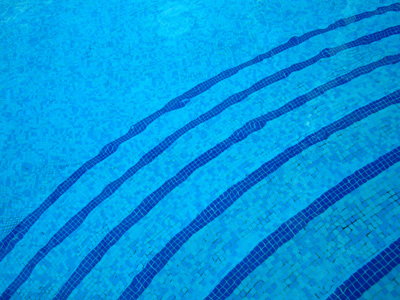To the nearest square metre, what is the area of a circular pool with a radius of 10m?

# Level 5-6 Shapes - Circles

Circles are one of the most common shapes. If you think about it, circles are just about everywhere - wheels, gears, pulleys - without circles transport and machinery would be almost impossible. So, with circles being so important, it won't surprise you to learn that they are one of the things you need to know about in KS3 Maths.

There are some special terms relating to circles which you will hopefully be familiar with - radius, diameter, circumference, arc, sector and of course π. In any circle the ratio of the distance around the curved perimeter to the distance across the circle through the centre is represented by π (the Greek letter pi) which has a value of just over 3 (3.1415926535.....)

It's a very good idea to get to know circles, their parts and the formulae associated with them. To help you, try this quiz all about circles. Take your time and read each question carefully before submitting your answers. That way, you won't end up going round and round and round! Good luck!

1.
A circular lawn has a 20 m radius. What is the approximate circumference of the lawn?
100 m
126 m
150 m
175 m
Using C = 2 π r, 2 x 3.141592 x 20, C = 125.66 m
2.
To the nearest square metre, what is the area of a circular pool with a radius of 10 m?
114 m2
214 m2
314 m2
414 m2
The radius = 10 m, so the calculation is: 3.141592 x 10 x 10 = 314.159 m2
3.
A round table has a diameter of 200 cm. What is the approximate circumference of the table?
286 cm
628 cm
1,256 cm
I need to know the radius first
Using C = 2 π r, 2 x 3.141592 x 100, C = 628.3184 cm. Remember to use radius and not diameter!
4.
What is the correct formula for the circumference of a circle?
C = 2πr
C = 2πd
C = 2π
C = 3πr
Circumference = 2 x 3.141592 x radius. This formula allows you to make intelligent estimates before you work out the real answer
5.
What can be calculated using πr2?
Area of a circle
Circumference of a circle
Diameter of a circle
Volume of a circle
Remember to use the radius of the circle and not the diameter
6.
What is the area of a circle with a radius of 7 cm (to one decimal place)?
53.9 cm
153.9 cm
315.9 cm
513.9 cm
The radius = 7 cm, so the calculation is: 3.141592 x 7 x 7 = 153.938 cm
7.
The distance measured around a circle is called the .......
brim
circumference
diameter
In circles we call this the circumference rather than the perimeter
8.
A line which passes through the centre of a circle and joins two points on its circumference is called a .......
centre line
circumference
diameter
The diameter is like 2 radii on the same bearing
9.
The area of a circular lawn is 628 m2. How far is it across to the nearest metre?
20
24
28
32
10.
A line which joins the centre of a circle to a point on the circumference is called a .......
centre line
diameter
half distance